I’ve already briefly done some work in the dataset in my tutorial for Logistic Regression – but never in entirety. I decided to re-evaluate utilizing Random Forest and submit to Kaggle. In this dataset, we’re utilizing a testing/training dataset of passengers on the Titanic in which we need to predict if passengers survived or not (1 or 0).

## Titanic Dataset – Kaggle Submission

You can view my Kaggle submissions here.

### Initial Imports

In :
```import pandas as pd
import numpy as np
import matplotlib.pyplot as plt
import seaborn as sns
%matplotlib inline
```

### Data

In :
```#Import training data
```
In :
```df_test.head()
```
Out:
PassengerId Pclass Name Sex Age SibSp Parch Ticket Fare Cabin Embarked
0 892 3 Kelly, Mr. James male 34.5 0 0 330911 7.8292 NaN Q
1 893 3 Wilkes, Mrs. James (Ellen Needs) female 47.0 1 0 363272 7.0000 NaN S
2 894 2 Myles, Mr. Thomas Francis male 62.0 0 0 240276 9.6875 NaN Q
3 895 3 Wirz, Mr. Albert male 27.0 0 0 315154 8.6625 NaN S
4 896 3 Hirvonen, Mrs. Alexander (Helga E Lindqvist) female 22.0 1 1 3101298 12.2875 NaN S
In :
```df_train.head()
```
Out:
PassengerId Survived Pclass Name Sex Age SibSp Parch Ticket Fare Cabin Embarked
0 1 0 3 Braund, Mr. Owen Harris male 22.0 1 0 A/5 21171 7.2500 NaN S
1 2 1 1 Cumings, Mrs. John Bradley (Florence Briggs Th… female 38.0 1 0 PC 17599 71.2833 C85 C
2 3 1 3 Heikkinen, Miss. Laina female 26.0 0 0 STON/O2. 3101282 7.9250 NaN S
3 4 1 1 Futrelle, Mrs. Jacques Heath (Lily May Peel) female 35.0 1 0 113803 53.1000 C123 S
4 5 0 3 Allen, Mr. William Henry male 35.0 0 0 373450 8.0500 NaN S

### Cleanse Missing Data

In :
```#We have some missing data here, so we're going to map out where NaN exists
#We might be able to take care of age, but cabin is probably too bad to save
sns.heatmap(df_train.isnull(),yticklabels=False,cbar=False,cmap='viridis')
```
Out:
`<matplotlib.axes._subplots.AxesSubplot at 0x16e436d6d08>`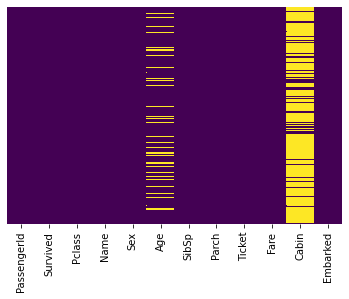Age has some missing values, and one way we could fix the problem would be to fill in the average age. However – we could take this a step further and grab the average age by passenger class. This could provide us a slightly more accurate value given that it appears age follows a pattern across classes.

Now – let’s take a quick look at the test dataset to see if we have the same issue.

In :
```#Test dataset
sns.heatmap(df_test.isnull(),yticklabels=False,cbar=False,cmap='viridis')
```
Out:
`<matplotlib.axes._subplots.AxesSubplot at 0x16e43ea2548>`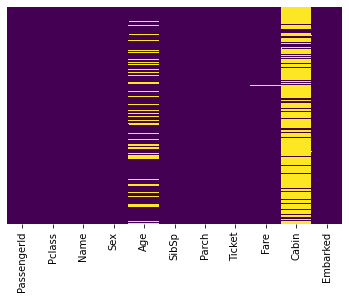Same problem here with Test, except that we do see one NULL in the Fare. Similar to age – we could replace this with an average, possibly by Class since Fare will most definitely be affected by that. Now let’s continue on with cleansing the Age.

In :
```#Determine the average ages of passengers by class
#In an attempt to fix the NaN for this column somewhat
sns.set_style('whitegrid')
plt.figure(figsize=(10,7))
sns.boxplot(x='Pclass',y='Age',data=df_train)
```
Out:
`<matplotlib.axes._subplots.AxesSubplot at 0x16e43e87588>`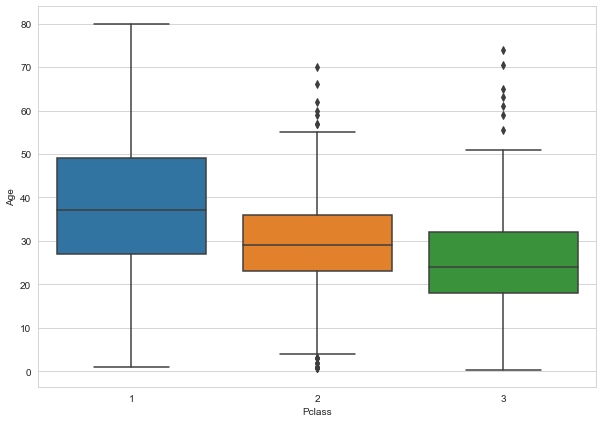#### Fix Age and Cabin in Both Train/Test

In :
```#Fill out the Age column with the average ages of passengers per class
def impute_age(cols):
Age = cols
Pclass = cols

if pd.isnull(Age):
if Pclass == 1:
return 37 #Return the avg age of passengers in the 1st class
elif Pclass == 2:
return 29 #2nd class
else:
return 24 #3rd class
else:
return Age

#Apply the function to the Age column
df_train['Age'] = df_train[['Age','Pclass']].apply(impute_age,axis=1)
df_test['Age'] = df_test[['Age','Pclass']].apply(impute_age,axis=1)

#Drop the cabin data
df_train.drop('Cabin',axis=1,inplace=True)
df_test.drop('Cabin',axis=1,inplace=True)
```
In :
```#Recheck the heatmap
#No more problems with Age
sns.heatmap(df_train.isnull(),yticklabels=False,cbar=False,cmap='viridis')
```
Out:
`<matplotlib.axes._subplots.AxesSubplot at 0x16e4415c8c8>`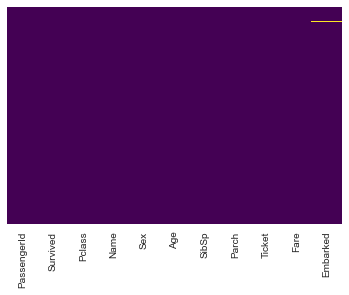In :
```sns.heatmap(df_test.isnull(),yticklabels=False,cbar=False,cmap='viridis')
```
Out:
`<matplotlib.axes._subplots.AxesSubplot at 0x16e43fe7d88>`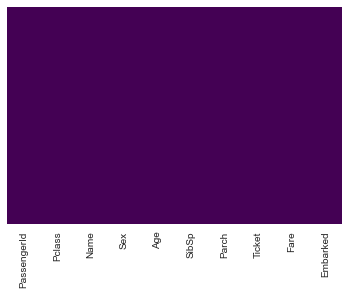This is a bit deceiving for Test – as we do still have a NaN Fare (as seen previously). We didn’t fix this yet, it’s just hidden a bit in this visualization.

#### Fix Fare in Test Dataset

In :
```df_test[df_test['Fare'].isnull() == True]
```
Out:
PassengerId Pclass Name Sex Age SibSp Parch Ticket Fare Embarked
152 1044 3 Storey, Mr. Thomas male 60.5 0 0 3701 NaN S

To fix this – let’s find the average fare for a 3rd class passenger.

In :
```df_test['Fare'][df_test['Pclass'] == 3].mean()
```
Out:
`12.459677880184334`
In :
```df_test['Fare'][df_test['PassengerId'] == 1044] = 12.45
```

#### Fix Embarked in Train Dataset

In :
```#Determine where most people in PClass = 1 embarked
#In an attempt to fix the NaN for this column somewhat
sns.set_style('whitegrid')
plt.figure(figsize=(10,7))
sns.countplot(x="Embarked", data=df_train[df_train['Pclass'] == 1])
```
Out:
`<matplotlib.axes._subplots.AxesSubplot at 0x16e440554c8>`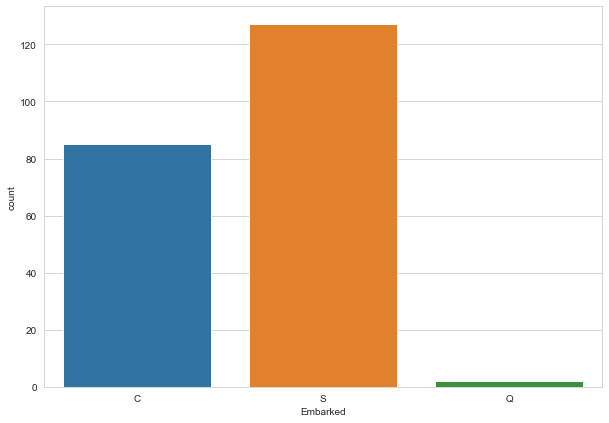In :
```df_train[df_train['Embarked'].isnull() == True]
```
Out:
PassengerId Survived Pclass Name Sex Age SibSp Parch Ticket Fare Embarked
61 62 1 1 Icard, Miss. Amelie female 38.0 0 0 113572 80.0 NaN
829 830 1 1 Stone, Mrs. George Nelson (Martha Evelyn) female 62.0 0 0 113572 80.0 NaN

Both of these rows are for customers inside of 1st class – so let’s see where most of those passengers embarked from.

In :
```#Determine where most people in PClass = 1 embarked
#In an attempt to fix the NaN for this column somewhat
sns.set_style('whitegrid')
plt.figure(figsize=(10,7))
sns.countplot(x="Embarked", data=df_train[df_train['Pclass'] == 1])
```
Out:
`<matplotlib.axes._subplots.AxesSubplot at 0x16e4411ef48>`Since most are from ‘S’ – we’ll make an executive decision here to set the others to ‘S’

In :
```df_train['Embarked'][df_train['Embarked'].isnull() == True] = 'S'
```
In :
```df_train[df_train['Embarked'].isnull() == True]
```
Out:
PassengerId Survived Pclass Name Sex Age SibSp Parch Ticket Fare Embarked

All fixed!

### Other data cleansing, Parse down dataset

In :
```#Transform male/female into numeric columns - we drop female since M/F are perfect predictors
#Transform embarked into numeric columns Q/S
sex_train = pd.get_dummies(df_train['Sex'],drop_first=True)
embark_train = pd.get_dummies(df_train['Embarked'],drop_first=True)

#Repeat for test dataset
sex_test = pd.get_dummies(df_test['Sex'],drop_first=True)
embark_test = pd.get_dummies(df_test['Embarked'],drop_first=True)
```
In :
```#Add the new columns to the dataset
df_train = pd.concat([df_train,sex_train,embark_train],axis=1)
df_test = pd.concat([df_test,sex_test,embark_test],axis=1)
```
In :
```#Drop the old Sex/Embarked columns, along with the other columns we can't use for predictions
#Save passenger ID's for submission
pass_id = df_test['PassengerId']

df_train.drop(['PassengerId','Sex','Embarked','Name','Ticket'],axis=1,inplace=True)
df_test.drop(['PassengerId','Sex','Embarked','Name','Ticket'],axis=1,inplace=True)
```
In :
```df_train.head()
```
Out:
Survived Pclass Age SibSp Parch Fare male Q S
0 0 3 22.0 1 0 7.2500 1 0 1
1 1 1 38.0 1 0 71.2833 0 0 0
2 1 3 26.0 0 0 7.9250 0 0 1
3 1 1 35.0 1 0 53.1000 0 0 1
4 0 3 35.0 0 0 8.0500 1 0 1
In :
```df_test.head()
```
Out:
Pclass Age SibSp Parch Fare male Q S
0 3 34.5 0 0 7.8292 1 1 0
1 3 47.0 1 0 7.0000 0 0 1
2 2 62.0 0 0 9.6875 1 1 0
3 3 27.0 0 0 8.6625 1 0 1
4 3 22.0 1 1 12.2875 0 0 1

### Run Machine Learning Algorithm

To start with I’m going to split the training set to get an idea of accuracy. I’ll be trying out Random Forests for my model.

In :
```#Assign variables
X = df_train.drop('Survived', axis=1)
y = df_train['Survived']
```
In :
```from sklearn.model_selection import train_test_split
```
In :
```#Choose the test size
#Test size = % of dataset allocated for testing (.3 = 30%)
#Random state = # of random splits
X_train, X_test, y_train, y_test = train_test_split(X, y, test_size=0.3, random_state=101)
```
In :
```from sklearn.ensemble import RandomForestClassifier
```
In :
```#Object, try out 200 estimators to start
rfc = RandomForestClassifier(n_estimators=200)
```
In :
```#Fit model
rfc.fit(X_train,y_train)
```
Out:
`RandomForestClassifier(n_estimators=200)`
In :
```#Form predictions
predictions = rfc.predict(X_test)
```
In :
```from sklearn.metrics import classification_report, confusion_matrix
```
In :
```#Print reports
print(confusion_matrix(y_test,predictions))
print(classification_report(y_test,predictions))
```
```[[130  24]
[ 32  82]]
precision    recall  f1-score   support

0       0.80      0.84      0.82       154
1       0.77      0.72      0.75       114

accuracy                           0.79       268
macro avg       0.79      0.78      0.78       268
weighted avg       0.79      0.79      0.79       268

```

Overall, it’s a pretty good model – but it’s still possible that we might be able to improve it a bit

### Try to improve with Randomized Searching (or Grid Searching)

Could have also utilized Grid Searching, but I wanted to try a large amount of parameters with low run-time.

In :
```#Imports
from sklearn.model_selection import RandomizedSearchCV
```
In :
```#Provide a dictionary of these values to test
param_grid = {'bootstrap': [True, False],
'max_depth': [10, 20, 30, 40, 50, 60, 70, 80, 90, 100, None],
'max_features': ['auto', 'sqrt'],
'min_samples_leaf': [1, 2, 4],
'min_samples_split': [2, 5, 10],
'n_estimators': [200, 400, 600, 800, 1000, 1200, 1400, 1600, 1800, 2000]}
```
In :
```#Instantiate object
grid = RandomizedSearchCV(estimator = RandomForestClassifier(), param_distributions = param_grid, n_iter = 100, cv = 3, verbose=2, random_state=42, n_jobs = -1)
```
In :
```#Fit to find the best combo of params
grid.fit(X_train,y_train)
```
```Fitting 3 folds for each of 100 candidates, totalling 300 fits
```
```[Parallel(n_jobs=-1)]: Using backend LokyBackend with 12 concurrent workers.
[Parallel(n_jobs=-1)]: Done  17 tasks      | elapsed:    7.5s
[Parallel(n_jobs=-1)]: Done 138 tasks      | elapsed:   31.3s
[Parallel(n_jobs=-1)]: Done 300 out of 300 | elapsed:  1.3min finished
```
Out:
```RandomizedSearchCV(cv=3, estimator=RandomForestClassifier(), n_iter=100,
n_jobs=-1,
param_distributions={'bootstrap': [True, False],
'max_depth': [10, 20, 30, 40, 50, 60,
70, 80, 90, 100, None],
'max_features': ['auto', 'sqrt'],
'min_samples_leaf': [1, 2, 4],
'min_samples_split': [2, 5, 10],
'n_estimators': [200, 400, 600, 800,
1000, 1200, 1400, 1600,
1800, 2000]},
random_state=42, verbose=2)```
In :
```#Show the best params to use
grid.best_params_
```
Out:
```{'n_estimators': 2000,
'min_samples_split': 2,
'min_samples_leaf': 2,
'max_features': 'auto',
'max_depth': 10,
'bootstrap': False}```
In :
```#New predictions
grid_predictions = grid.predict(X_test)
```
In :
```#Print reports
print(confusion_matrix(y_test,grid_predictions))
print(classification_report(y_test,grid_predictions))
```
```[[138  16]
[ 32  82]]
precision    recall  f1-score   support

0       0.81      0.90      0.85       154
1       0.84      0.72      0.77       114

accuracy                           0.82       268
macro avg       0.82      0.81      0.81       268
weighted avg       0.82      0.82      0.82       268

```

We actually did see a slight improvement here over the original model 🙂

### Re-train on entire (un-split) training dataset

Now that we’ve gotten the “best” paramaters, we’ll try to re-train utilizing the entire training dataset before we run final predictions.

Out of curiousity – I tried skipping this set and submitting without re-training on the full set, and I got a score of 0.76 from Kaggle (meaning 76% of predictions were correct). So let’s see if this makes a big difference…

In :
```#Object, try out 200 estimators to start
rfc_full = RandomForestClassifier(n_estimators=2000,
min_samples_split=2,
min_samples_leaf=2,
max_features='auto',
max_depth=10,
bootstrap=False)

#Fit model
rfc_full.fit(X,y)
```
Out:
```RandomForestClassifier(bootstrap=False, max_depth=10, min_samples_leaf=2,
n_estimators=2000)```

### Run finalized model on Test dataset

In :
```#Run on the test dataframe
full_predictions = rfc_full.predict(df_test)
```
In :
```full_predictions
```
Out:
```array([0, 0, 0, 0, 1, 0, 0, 0, 1, 0, 0, 0, 1, 0, 1, 1, 0, 0, 0, 1, 1, 1,
1, 1, 1, 0, 1, 0, 1, 0, 0, 0, 1, 0, 1, 0, 0, 0, 0, 1, 0, 1, 0, 1,
1, 0, 0, 0, 1, 1, 0, 0, 1, 1, 0, 0, 0, 0, 0, 1, 0, 0, 0, 1, 1, 1,
1, 0, 0, 1, 1, 0, 0, 0, 1, 0, 0, 1, 0, 1, 1, 0, 0, 0, 0, 0, 1, 0,
1, 1, 0, 0, 1, 0, 0, 0, 1, 0, 0, 0, 1, 0, 0, 0, 0, 0, 0, 0, 0, 0,
0, 1, 1, 0, 1, 0, 0, 1, 0, 1, 1, 0, 1, 0, 0, 1, 0, 1, 0, 0, 0, 0,
0, 0, 0, 0, 0, 0, 0, 0, 0, 1, 0, 0, 1, 0, 0, 0, 1, 0, 1, 0, 0, 1,
0, 0, 1, 0, 1, 1, 1, 1, 1, 0, 0, 0, 0, 0, 1, 0, 0, 0, 0, 0, 0, 1,
1, 1, 1, 1, 0, 0, 1, 0, 1, 0, 1, 0, 0, 0, 0, 0, 1, 0, 1, 0, 1, 0,
0, 0, 1, 1, 0, 1, 0, 0, 0, 0, 1, 0, 0, 0, 0, 1, 0, 0, 1, 0, 1, 0,
1, 0, 1, 0, 1, 1, 0, 1, 0, 0, 0, 1, 0, 0, 1, 0, 1, 0, 1, 1, 1, 1,
0, 0, 0, 0, 1, 0, 1, 0, 1, 0, 0, 0, 0, 0, 0, 0, 1, 0, 0, 0, 1, 1,
0, 0, 0, 0, 0, 0, 0, 0, 1, 1, 0, 1, 0, 0, 0, 0, 0, 1, 1, 0, 1, 0,
0, 0, 0, 0, 0, 0, 0, 0, 0, 0, 1, 0, 0, 0, 0, 0, 0, 0, 1, 1, 1, 1,
0, 0, 0, 0, 0, 0, 1, 1, 0, 0, 0, 0, 0, 0, 0, 1, 1, 0, 1, 0, 0, 0,
1, 0, 0, 1, 0, 0, 0, 0, 0, 0, 0, 0, 0, 1, 0, 1, 0, 1, 0, 1, 1, 0,
0, 0, 1, 0, 1, 0, 0, 0, 0, 1, 1, 0, 1, 0, 0, 0, 1, 0, 0, 1, 0, 0,
1, 1, 0, 0, 0, 0, 0, 0, 1, 1, 0, 1, 0, 0, 0, 0, 0, 1, 0, 0, 0, 1,
0, 1, 0, 0, 1, 0, 1, 0, 0, 0, 0, 0, 1, 1, 1, 1, 0, 0, 1, 0, 0, 1],
dtype=int64)```
In :
```#Merge into final dataframe
pred_df = pd.DataFrame(full_predictions)

#Drop indexes (can cause NaN when using Concat if you don't do this beforehand)
pred_df.reset_index(drop=True, inplace=True)
pass_id.reset_index(drop=True, inplace=True)

submission = pd.concat([pass_id.iloc[0:],pred_df], axis=1)
submission.columns=['PassengerId','Survived']
submission.to_csv('submission.csv', index = False)
```
In :
```submission.head()
```
Out:
PassengerId Survived
0 892 0
1 893 0
2 894 0
3 895 0
4 896 1

Submitting this to Kaggle – things fall in line largely with the performance shown in the training dataset. I’m getting a score of 0.77751, meaning that I’ve predicted roughly 77-78% entries correctly. We could certainly continue on, testing and tuning different models to get improved performance

You can view my Kaggle submissions here.

WordPress conversion from Titanic Dataset – Kaggle Submission.ipynb by nb2wp v0.3.1